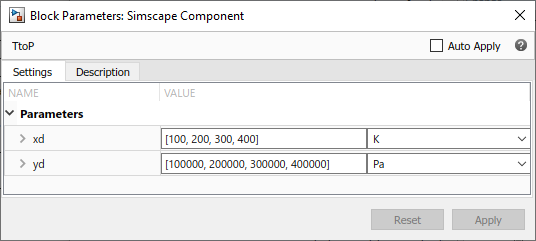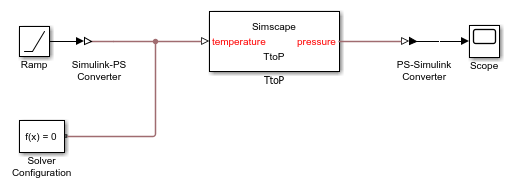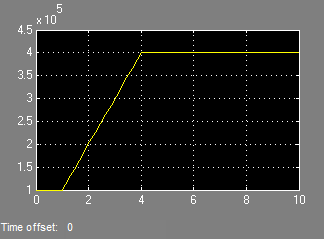## Using Lookup Tables in Equations

You can use the `tablelookup` function in the `equations` section of the Simscape™ file to interpolate input values based on a set of data points in a one-dimensional, two-dimensional, or three-dimensional table. This functionality is similar to that of the Simulink® and Simscape Lookup Table blocks. It allows you to incorporate table-driven modeling directly in your custom block, without the need of connecting an external Lookup Table block to your model.

The following example implements mapping temperature to pressure using a one-dimensional lookup table.

```component TtoP inputs u = {0,'K'}; % temperature end outputs y = {0,'Pa'}; % pressure end parameters (Size=variable) xd = {[100 200 300 400] 'K'}; yd = {[1e5 2e5 3e5 4e5] 'Pa'}; end equations y == tablelookup(xd,yd,u,interpolation=linear,extrapolation=nearest); end end ```

`xd` and `yd` are declared as variable-size parameters with units. This enables the block users to provide their own data sets when the component is converted to a custom block, and also to select commensurate units from the drop-downs in the custom block dialog box. The next illustration shows the dialog box of the custom block generated from this component.Note

Currently, you cannot use variable-size parameters in the `equations` section outside of the `tablelookup` function.

To avoid repeating the same variable-size parameter declarations in each component that needs to use them in its `tablelookup` function, you can declare variable-size domain parameters and propagate them to components for interpolation purposes. For more information, see Propagation of Domain Parameters.

The following rules apply to the one-dimensional arrays `xd` and `yd`:

• The two arrays must be of the same size.

• For smooth interpolation, each array must contain at least three values. For linear interpolation, two values are sufficient.

• The `xd` values must be strictly monotonic, either increasing or decreasing.

The TtoP component uses linear interpolation for values within the table range, but outputs the nearest value of `yd` for out-of-range input values. The following illustration shows a block diagram, where the custom TtoP block is used with a linear input signal changing from 0 to 1000, and the resulting output.See the `tablelookup` reference page for syntax specifics and more examples.